# AC reactive circuit calculations

## Electricity and Electronics

• #### Question 1

Determine the input frequency necessary to give the output voltage a phase shift of 75$^{o}$: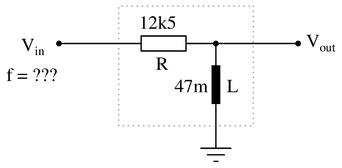Also, write an equation that solves for frequency ($f$), given all the other variables ($R$, $L$, and phase angle $\theta$).

Reveal answer
• #### Question 2

An AC electric motor under load can be considered as a parallel combination of resistance and inductance:Calculate the equivalent inductance ($L_{eq}$) if the measured source current is 27.5 amps and the motor’s equivalent resistance ($R_{eq}$) is 11.2 $\Omega$.

Reveal answer
• #### Question 3

An AC electric motor under load can be considered as a parallel combination of resistance and inductance:Calculate the current necessary to power this motor if the equivalent resistance and inductance is 20 $\Omega$ and 238 mH, respectively.

Reveal answer
• #### Question 4

Calculate the individual currents through the inductor and through the resistor, the total current, and the total circuit impedance:Also, draw a phasor diagram showing how the individual component currents relate to the total current.

Reveal answer
• #### Question 5

Calculate the total impedances (complete with phase angles) for each of the following capacitor-resistor circuits:Reveal answer
• #### Question 6

Calculate the total impedances (complete with phase angles) for each of the following inductor-resistor circuits:Reveal answer
• #### Question 7

Is this circuit’s overall behavior capacitive or inductive? In other words, from the perspective of the AC voltage source, does it appear’’ as though a capacitor is being powered, or an inductor?Now, suppose we take these same components and re-connect them in parallel rather than series. Does this change the circuit’s overall appearance’’ to the source? Does the source now see’’ an equivalent capacitor or an equivalent inductor? Explain your answer.{\bullet} Which component dominates’’ the behavior of a series LC circuit, the one with the least reactance or the one with the greatest reactance?
{\bullet} Which component dominates’’ the behavior of a parallel LC circuit, the one with the least reactance or the one with the greatest reactance?

Reveal answer
• #### Question 8

Calculate the voltage dropped across the inductor, the capacitor, and the 8-ohm speaker in this sound system at the following frequencies, given a constant source voltage of 15 volts: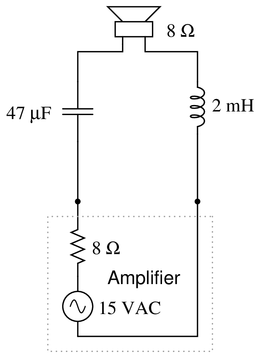{\bullet} $f =$ 200 Hz
{\bullet} $f =$ 550 Hz
{\bullet} $f =$ 900 Hz

Regard the speaker as nothing more than an 8-ohm resistor.

{\bullet} As part of an audio system, would this LC network tend to emphasize the {\it bass}, {\it treble}, or {\it mid-range} tones?

Reveal answer
• #### Question 9

Write an equation that solves for the impedance of this series circuit. The equation need not solve for the phase angle between voltage and current, but merely provide a scalar figure for impedance (in ohms):Reveal answer
• #### Question 10

Determine the necessary resistor value to give the output voltage a phase shift of $-64^{o}$: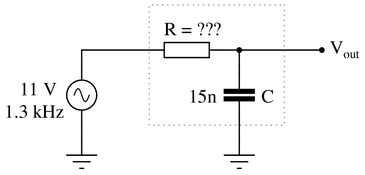Also, write an equation that solves for this resistance value ($R$), given all the other variables ($f$, $C$, and phase angle $\theta$).

Reveal answer
• #### Question 11

Determine the necessary resistor value to give the output voltage a phase shift of 58$^{o}$: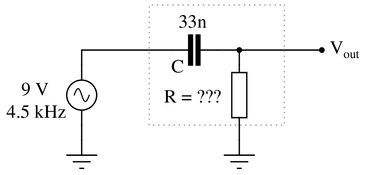Also, write an equation that solves for this resistance value ($R$), given all the other variables ($f$, $C$, and phase angle $\theta$).

Reveal answer
• #### Question 12

Determine the input frequency necessary to give the output voltage a phase shift of 25$^{o}$: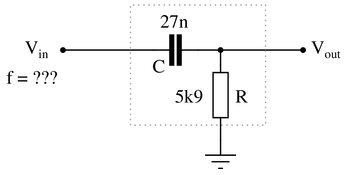Also, write an equation that solves for frequency ($f$), given all the other variables ($R$, $C$, and phase angle $\theta$).

Reveal answer
• #### Question 13

Determine the input frequency necessary to give the output voltage a phase shift of $-40^{o}$: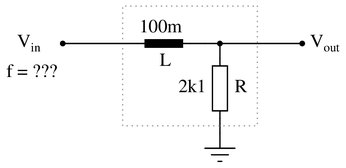Also, write an equation that solves for frequency ($f$), given all the other variables ($R$, $L$, and phase angle $\theta$).

Reveal answer
• #### Question 14

Calculate the total impedance offered by these two inductors to a sinusoidal signal with a frequency of 60 Hz: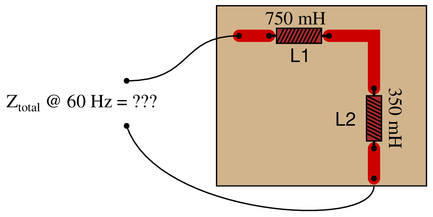Show your work using two different problem-solving strategies:

{\bullet} Calculating total inductance ($L_{total}$) first, then total impedance ($Z_{total}$).
{\bullet} Calculating individual impedances first ($Z_{L1}$ and $Z_{L2}$), then total impedance ($Z_{total}$).

Do these two strategies yield the same total impedance value? Why or why not?

Reveal answer
• #### Question 15

Determine the input frequency necessary to give the output voltage a phase shift of $-25^{o}$: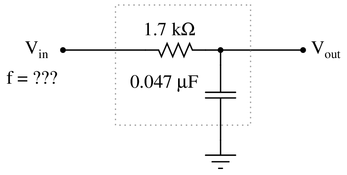Reveal answer
• #### Question 16

Determine the input frequency necessary to give the output voltage a phase shift of 40$^{o}$: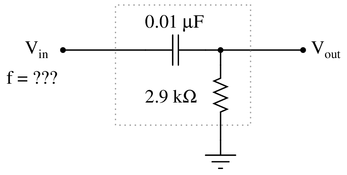Reveal answer
• #### Question 17

A student is asked to calculate the phase shift for the following circuit’s output voltage, relative to the phase of the source voltage:He recognizes this as a series circuit, and therefore realizes that a right triangle would be appropriate for representing component impedances and component voltage drops (because both impedance and voltage are quantities that add in series, and the triangle represents phasor addition):The problem now is, which angle does the student solve for in order to find the phase shift of $V_{out}$? The triangle contains two angles besides the 90$^{o}$ angle, $\theta$ and $\Phi$. Which one represents the output phase shift, and more importantly, {\it why}?

Reveal answer
• #### Question 18

Determine the total current and all voltage drops in this circuit, stating your answers the way a multimeter would register them: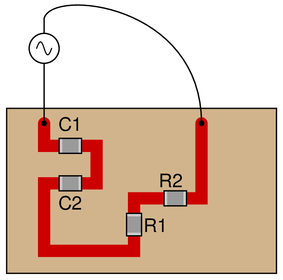{\bullet} $C_1 = 125 \hbox{ pF}$
{\bullet} $C_2 = 71 \hbox{ pF}$
{\bullet} $R_1 = 6.8 \hbox{ k}\Omega$
{\bullet} $R_2 = 1.2 \hbox{ k}\Omega$
{\bullet} $V_{supply} = 20 \hbox{ V RMS}$
{\bullet} $f_{supply} = 950 \hbox{ kHz}$

Also, calculate the phase angle ($\theta$) between voltage and current in this circuit, and explain where and how you would connect an oscilloscope to measure that phase shift.

Reveal answer
• #### Question 19

Solve for all voltages and currents in this series RC circuit, and also calculate the phase angle of the total impedance:Reveal answer
• #### Question 20

Solve for all voltages and currents in this series RC circuit:Reveal answer
• #### Question 21

Solve for all voltages and currents in this series LR circuit, and also calculate the phase angle of the total impedance:Reveal answer
• #### Question 22

Due to the effects of a changing electric field on the dielectric of a capacitor, some energy is dissipated in capacitors subjected to AC. Generally, this is not very much, but it is there. This dissipative behavior is typically modeled as a series-connected resistance: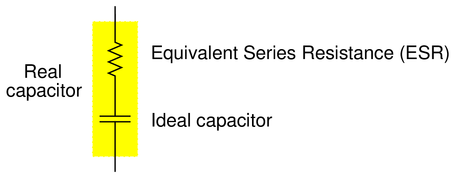Calculate the magnitude and phase shift of the current through this capacitor, taking into consideration its equivalent series resistance (ESR):Compare this against the magnitude and phase shift of the current for an ideal 0.22 $\mu$F capacitor.

Reveal answer
• #### Question 23

Which component, the resistor or the capacitor, will drop more voltage in this circuit?Also, calculate the total impedance ($Z_{total}$) of this circuit, expressing it in both rectangular and polar forms.

Reveal answer
• #### Question 24

Calculate all voltages and currents in this circuit, as well as the total impedance:Reveal answer
• #### Question 25

Use the impedance triangle’’ to calculate the necessary reactance of this series combination of resistance ($R$) and inductive reactance ($X$) to produce the desired total impedance of 145 $\Omega$:Explain what equation(s) you use to calculate $X$, and the algebra necessary to achieve this result from a more common formula.

Reveal answer
• #### Question 26

Use the impedance triangle’’ to calculate the impedance of this series combination of resistance ($R$) and inductive reactance ($X$):Explain what equation(s) you use to calculate $Z$.

Reveal answer

### Related Content

• Share

Published under the terms and conditions of the Creative Commons Attribution License

0 Comments